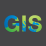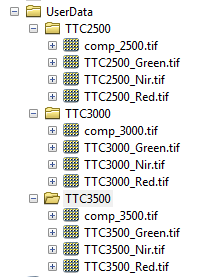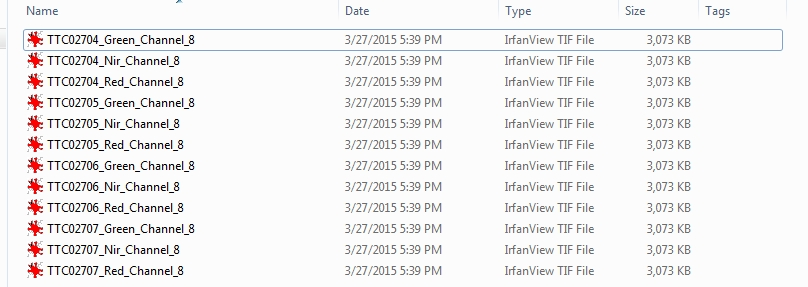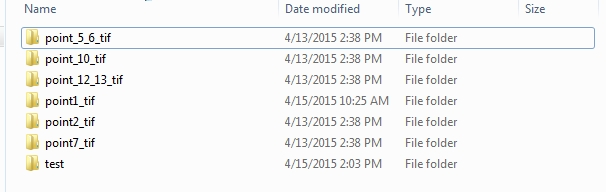# Python Script to iterate numerous, single-band rasters for arcpy.CompositeBands

4904
11
04-14-2015 02:42 PMNew Contributor

I have a large collection of single-band geotiffs (NIR, Green, and Red) that I would like to composite using a Python script. The goal is to iterate through multiple folders in a main directory, identify each tiff as band1, band2, band3 to input into CompositeBands_management, and do this for every raster scene. To clarify, each image number, i.e. scene, has three single-band tiffs associated. For example, the naming convention is: TTC2500_Green_Channel_8.TIF where TTC2500 is the image number.

So far, my script can go through each folder and identify the specific bands based on the filename but I am having difficulty bringing them into the tool. The attached script has the Composite tool commented out but the result is a printed list of each geotiff. Any thoughts on how to move from here into an automated processing method to output new false color composite geotiffs?

```import arcpy
import os
from arcpy.sa import *
from arcpy import env

env.workspace=(r"C:\Users\cc\Desktop\tcamPractice\point1_tif")
env.overwriteOutput = True

outws=(r"C:\Users\cc\Desktop\tcamPractice\false")
for root, dirs, files in os.walk(r"C:\Users\cc\Desktop\tcamPractice\point1_tif"):
for raster in files:
rasterlist=arcpy.ListRasters("*", "TIF")
outraster=outws+"comp.tif"
if raster.find("Green")>0:
band1=raster
print band1
elif raster.find("Red")>0:
band2=raster
print band2
elif raster.find("Nir")>0:
band3=raster
print band3
arcpy.CompositeBands_management(raster, outraster)

```
Tags (4)
1 Solution

Accepted SolutionsbyEsri Esteemed Contributor

The below should work.  You can create a list of all the flights, i.e. TCC2500.  Then, remove the duplicates from this list.  Next, you can iterate through this list and create the composite based on the flight.

```import arcpy
from arcpy import env
env.overwriteOutput = 1

workspace = r"C:\temp\python\UserData"

#create list
list = []

#populate list with flight, i.e. TTC2500
walk = arcpy.da.Walk(workspace, datatype="RasterDataset", type="TIF")
for dirpath, dirnames, filenames in walk:
for raster in filenames:
list.append(raster.split("_"))

#remove duplicates from list
list = dict.fromkeys(list)
list = list.keys()

for n in list:
walk = arcpy.da.Walk(workspace, datatype="RasterDataset", type="TIF")
for dirpath, dirnames, filenames in walk:
env.workspace = dirpath
for raster in filenames:
if n + "_Green" in raster:
green = env.workspace + "\\" + raster
elif n + "_Red" in raster:
red = env.workspace + "\\" + raster
elif n + "_Nir" in raster:
nir = env.workspace + "\\" + raster
if green != '' and red != '' and nir != '':
compBands = '"' + nir + ';' + green + ';' + red + ';"'
compRasterName = "comp_" + str(n[3:]) + ".tif"
outputRaster = env.workspace + "\\" + compRasterName
arcpy.CompositeBands_management(compBands, outputRaster)```
11 RepliesbyEsri Esteemed Contributor

Hi Casey,

You could use something along the lines of the below code:

```import arcpy
from arcpy import env
env.overwriteOutput = 1

workspace = r"C:\temp\python\UserData"

walk = arcpy.da.Walk(workspace, datatype="RasterDataset", type="TIF")
for dirpath, dirnames, filenames in walk:
env.workspace = dirpath
green = ''
red = ''
nir = ''
for raster in filenames:
if "green" in raster.lower():
green = env.workspace + "\\" + raster
elif "red" in raster.lower():
red = env.workspace + "\\" + raster
elif "nir" in raster.lower():
nir = env.workspace + "\\" + raster
if green != '' and red != '' and nir != '':
compBands = '"' + nir + ';' + green + ';' + red + ';"'
outputRaster = env.workspace + "\\comp.tif"
arcpy.CompositeBands_management(compBands, outputRaster)```New Contributor

Thanks Jake. This allows me to specify the tiffs to be used as either Nir, red, or green band in the CompositeBands tool. Defining those input objects will help with inputs for other tools too. My next goal is to modify this code to iterate through all images in the directory and create a unique composite band for each scene.

Is there a way to manipulate image number from the filename and append it to each composite image? For example, the Nir, red, and green image from TTC2500_Nir_Channel_8.TIF to comp_2500.TIF, where 2500 is the image number?byEsri Esteemed Contributor

Do all of the TIFFs follow the same naming convention, i.e. three characters followed by the image number?New Contributor

That's right. For example:

TTC2500_Nir_Channel_8., TTC2500_Red_Channel_8.TIF, TTC2500_Green_Channel_8.TIF

TTC2501_Nir_Channel_8.TIF, TTC2501_Red_Channel_8.TIF, TTC2501_Green_Channel_8.TIF

TTC2502_Nir_Channel_8.TIF, TTC2502_Red_Channel_8.TIF, TTC2502_Green_Channel_8.TIFbyEsri Esteemed Contributor

You can update a couple lines of code.  Try the following:

```if green != '' and red != '' and nir != '':
compBands = '"' + nir + ';' + green + ';' + red + ';"'
compRasterName = "comp_" + raster.split("_")[3:] + ".tif"
outputRaster = env.workspace + "\\" + compRasterName
arcpy.CompositeBands_management(compBands, outputRaster)```New Contributor

Excellent, renaming the output works like a charm. I can see where this coding technique to can be frequently used in Arc tools. I am still having a problem with the iteration. After running the script there is only one resulting composite image. It appears to iterate through all of the images because the result is named for the last image in the list. Would a save or copy raster tool help in this case to output each composite or would  an additional loop be necessary? Thanks by the way for you help!byEsri Esteemed Contributor

I could not reproduce this.  Here is how I have my data structured for testing.  You will see the composite TIFFs created correctly in each directory after the script is executed.New Contributor

I see. Thanks for showing your organization. Each set of scenes corresponds to a particular flight path. My organization was a little different in that I had folders populated with multiple scenes as opposed to each scene being in a folder. for example:This is the case for multiple folders in a main directory:I could simply write a code that IDs each image number, places all three images to an individual folder, and then run the composite but I'd rather be able to simply specify the main directory where each point folder is located and have the composites produced for every scene in each folder. Not sure if my description makes sense but I will continue to test it out this afternoon and post something if I get it working. Any suggestions?byEsri Esteemed Contributor

The below should work.  You can create a list of all the flights, i.e. TCC2500.  Then, remove the duplicates from this list.  Next, you can iterate through this list and create the composite based on the flight.

```import arcpy
from arcpy import env
env.overwriteOutput = 1

workspace = r"C:\temp\python\UserData"

#create list
list = []

#populate list with flight, i.e. TTC2500
walk = arcpy.da.Walk(workspace, datatype="RasterDataset", type="TIF")
for dirpath, dirnames, filenames in walk:
for raster in filenames:
list.append(raster.split("_"))

#remove duplicates from list
list = dict.fromkeys(list)
list = list.keys()

for n in list:
walk = arcpy.da.Walk(workspace, datatype="RasterDataset", type="TIF")
for dirpath, dirnames, filenames in walk:
env.workspace = dirpath
for raster in filenames:
if n + "_Green" in raster:
green = env.workspace + "\\" + raster
elif n + "_Red" in raster:
red = env.workspace + "\\" + raster
elif n + "_Nir" in raster:
nir = env.workspace + "\\" + raster
if green != '' and red != '' and nir != '':
compBands = '"' + nir + ';' + green + ';' + red + ';"'
compRasterName = "comp_" + str(n[3:]) + ".tif"
outputRaster = env.workspace + "\\" + compRasterName
arcpy.CompositeBands_management(compBands, outputRaster)```